# Texas Go Math Grade 5 Unit 4 Answer Key Geometry and Measurement

Refer to our Texas Go Math Grade 5 Answer Key Pdf to score good marks in the exams. Test yourself by practicing the problems from Texas Go Math Grade 5 Unit 4 Answer Key Geometry and Measurement.

## Texas Go Math Grade 5 Unit 4 Answer Key Geometry and Measurement

Show What You Know

Check your understanding of important skills.

Measure Length to the Nearest Inch
Use an inch ruler. Measure the length to the nearest inch.Question 1.Question 2.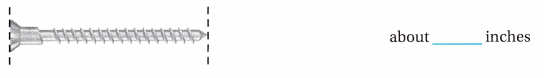Classify and Measure Angles

Classify the angle. Write acute, right, or obtuse.

Question 3.The given figure is:Now,
We know that,
If the angle is 90°, then it is a “Right angle”
If the angle is less than 90°, then it is “Acute angle”
If the angle is greater than 90°, then it is “Obtuse angle”
Hence, from the above,
We can conclude that
The given figure is a “Right angle”

Question 4.The given figure is: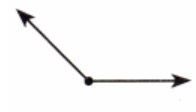Now,
We know that,
If the angle is 90°, then it is a “Right angle”
If the angle is less than 90°, then it is “Acute angle”
If the angle is greater than 90°, then it is “Obtuse angle”
Hence, from the above,
We can conclude that
The given figure is an “Obtuse angle”

Question 5.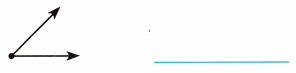The given figure is: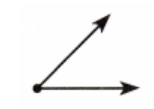Now,
We know that,
If the angle is 90°, then it is a “Right angle”
If the angle is less than 90°, then it is “Acute angle”
If the angle is greater than 90°, then it is “Obtuse angle”
Hence, from the above,
We can conclude that
The given figure is an “Acute angle”

Question 6.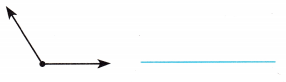The given figure is:Now,
We know that,
If the angle is 90°, then it is a “Right angle”
If the angle is less than 90°, then it is “Acute angle”
If the angle is greater than 90°, then it is “Obtuse angle”
Hence, from the above,
We can conclude that
The given figure is an “Obtuse angle”

Write intersecting, parallel, or perpendicular to describe the numbered sides.

Question 7.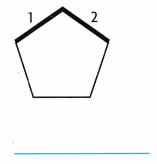The given figure is:Now,
We know that,
When two lines intersect and they don’t have an intersecting angle of 90°, they are “Intersecting Lines”
When two lines do not intersect, they are “parallel lines”
When two lines intersect and  have an intersecting angle of 90°, they are “Perpendicular Lines”
Hence, from the above,
We can conclude that
The numbered sides are “Perpendicular lines”

Question 8.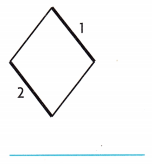The given figure is: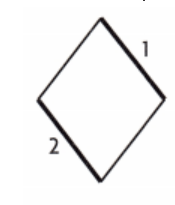Now,
We know that,
When two lines intersect and they don’t have an intersecting angle of 90°, they are “Intersecting Lines”
When two lines do not intersect, they are “parallel lines”
When two lines intersect and  have an intersecting angle of 90°, they are “Perpendicular Lines”
Hence, from the above,
We can conclude that
The numbered sides are “Parallel lines”

Question 9.The given figure is: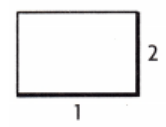Now,
We know that,
When two lines intersect and they don’t have an intersecting angle of 90°, they are “Intersecting Lines”
When two lines do not intersect, they are “parallel lines”
When two lines intersect and  have an intersecting angle of 90°, they are “Perpendicular Lines”
Hence, from the above,
We can conclude that
The numbered sides are “Perpendicular lines”

Vocabulary Builder

Visualize It
Sort the checked words into the circle map.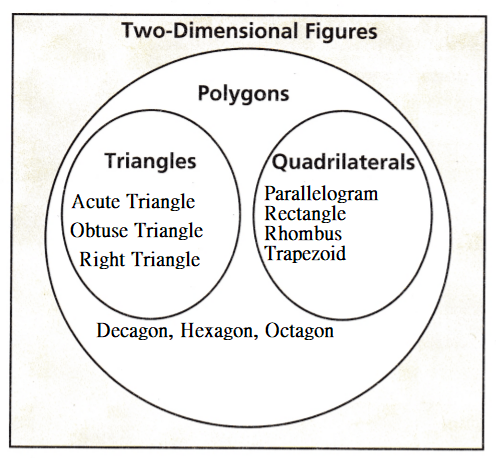Understand Vocabulary

Complete the sentences using the preview words.

Question 1.
The pair of numbers used to locate points on a grid is an ____.
We know that,
The pair of numbers used to locate points on a grid is an “ordered Pair”
Hence, from the above,
We can conclude that
The word that is suitable for the given statement is: Ordered Pair

Question 2.
All sides and all angles are ___ in a regular polygon.
We know that,
All sides and all angles are equal in a regular polygon.
Hence, from the above,
We can conclude that
The word that is suitable for the given statement is: Equal

Question 3.
The first number in an ordered pair is the __ and the second number in an ordered pair is the ___
We know that,
The first number in an ordered pair is the “X-Coordinate” and the second number in an ordered pair is the “Y-Coordinate”
Hence, from the above,
We can conclude that
The words that are suitable for the given statement is: X-Coordinate and Y-Coordinate

Question 4.
A cube with length, width, and height of 1 unit is a ____
We know that,
A cube with length, width, and height of 1 unit is a “Unit Cube”
Hence, from the above,
We can conclude that
The word that is suitable for the given statement is: Unit Cube

Question 5.
All three sides are the same length in a(n) _____
We know that,
All three sides are the same length in an “Equilateral Triangle”
Hence, from the above,
We can conclude that
The word that is suitable for the given statement is: Equilateral Triangle

Question 6.
The point, (0, 0), also called the ____, is where the x-axis and the y-axis intersect.
We know that,
The point, (0, 0), also called the “Origin”, is where the x-axis and the y-axis intersect.
Hence, from the above,
We can conclude that
The word that is suitable for the given statement is: Origin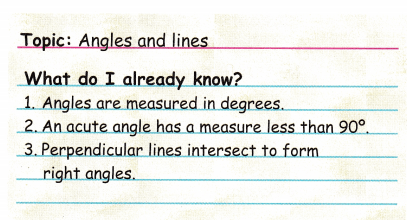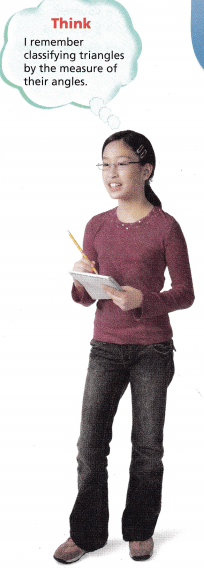Before beginning a module on geometry, Lauren lists three things she already knows.

Writing Think about all the words in your math vocabulary that relate to angles and lines, for example, right angle, parallel lines, and obtuse angle. Work with a partner to write five more things you know about the topic.
There are three types of triangles based on the type of angle. They are:
a. Acute Triangle
b. Right Triangle
c. Obtuse Triangle
There are three types of triangles based on the length of the sides. They are:
a. Equilateral triangle
b. Isosceles triangle
c. Scalene Triangle
Theere are three types of lines based on the intersecting points. They are:
a. Intersecting lines
b. Parallel lines
c. Perepndicular lines

Three-in-a-Row

The object of the Game
Identify and draw geometric figures.

Materials

• Counters
• 3-by-3 Grid
• Geometry Cards

SetUp
Give each player a 3-by-3 grid.
Players shuffle the Geometry Cards
and place them face down in a stack.
Number of Players 2—3

How to Play

1. Each player sketches the following geometric figures in any space on their grid: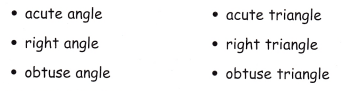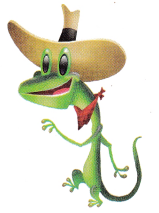2. Students take turns turning over the top card from the stack.
3. Students place a counter over the drawing on their grid that matches the Geometry Card.
4. The first player to get three in a row horizontally, vertically, or diagonally is the winner.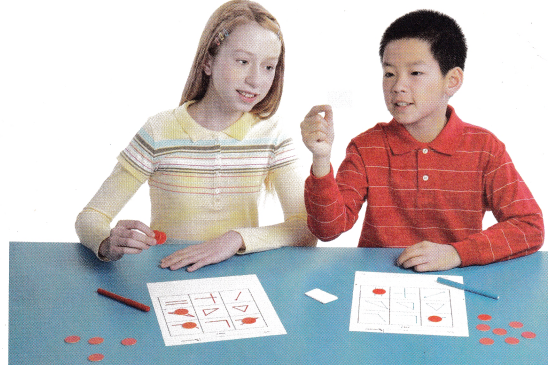Scroll to Top